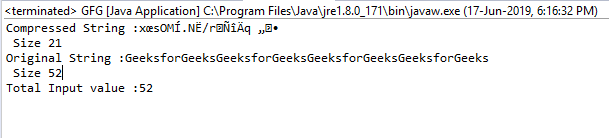Related Articles

# Deflater getTotalIn() function in Java with examples

• Last Updated : 24 Jun, 2019

The getTotalIn() function of the Deflater class in java.util.zip returns the total number of uncompressed bytes input provided till now.

Function Signature:

```public int getTotalIn()
```

Syntax:

```d.getTotalIn();
```

Parameter: The function requires no parameter

Return Type: The function returns an int value which is the total number of uncompressed bytes input.

Exception: The function does not throw any exception

Example 1:

 `// Java program to describe the use``// of getTotalIn() function`` ` `import` `java.util.zip.*;``import` `java.io.UnsupportedEncodingException;`` ` `class` `GFG {``    ``public` `static` `void` `main(String args[])``        ``throws` `UnsupportedEncodingException``    ``{``        ``// deflater``        ``Deflater d = ``new` `Deflater();`` ` `        ``// get the text``        ``String pattern = ``"GeeksforGeeks"``, text = ``""``;`` ` `        ``// generate the text``        ``for` `(``int` `i = ``0``; i < ``4``; i++)``            ``text += pattern;`` ` `        ``// set the input for deflator``        ``d.setInput(text.getBytes(``"UTF-8"``));`` ` `        ``// finish``        ``d.finish();`` ` `        ``// output bytes``        ``byte` `output[] = ``new` `byte``[``1024``];`` ` `        ``// compress the data``        ``int` `size = d.deflate(output);`` ` `        ``// compressed String``        ``System.out.println(``"Compressed String :"``                           ``+ ``new` `String(output)``                           ``+ ``"\n Size "` `+ size);`` ` `        ``// original String``        ``System.out.println(``"Original String :"``                           ``+ text + ``"\n Size "``                           ``+ text.length());`` ` `        ``// get the total number of uncompressed``        ``// bytes input so far``        ``System.out.println(``"Total Input value :"``                           ``+ d.getTotalIn());`` ` `        ``// end``        ``d.end();``    ``}``}`

Output:

```Compressed String :x?sOM?.N?/r???q??
Size 21
Original String :GeeksforGeeksGeeksforGeeksGeeksforGeeksGeeksforGeeks
Size 52
Total In value :52
```Note: The problem arises when the length of the input is greater than Integer.MAX_VALUE. Then the result may overflow, we should use getBytesRead() function instead

Attention reader! Don’t stop learning now. Get hold of all the important Java Foundation and Collections concepts with the Fundamentals of Java and Java Collections Course at a student-friendly price and become industry ready. To complete your preparation from learning a language to DS Algo and many more,  please refer Complete Interview Preparation Course.

My Personal Notes arrow_drop_up